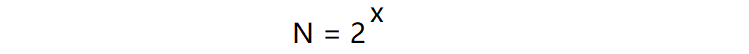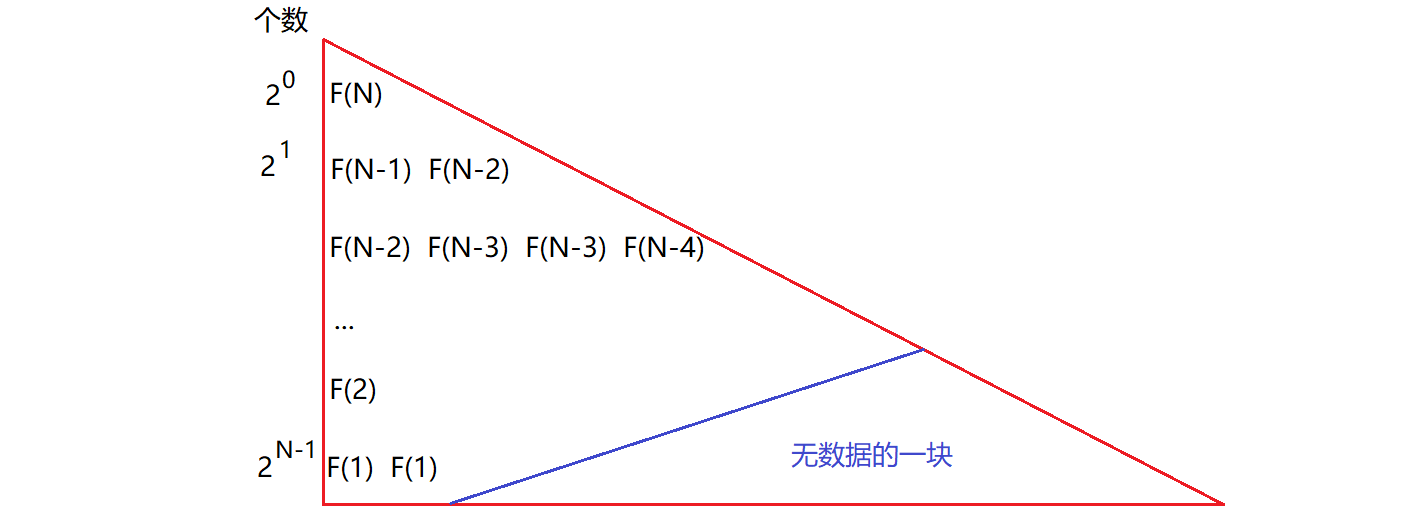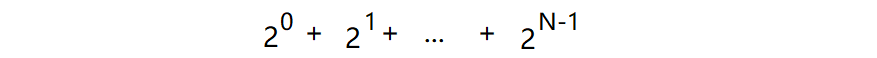# 大O的渐进表示法规则

1、所有常数都用常数1表示。
2、只保留最高阶项。
3、如果最高阶项存在且不是1，则去除与这个项的系数，得到的结果就是大O阶。

# 时间复杂度

//计算Func1的时间复杂度
void Func1(int N)
{
int count = 0;
for (int i = 0; i < 2 * N; i++)
{
for (int j = 0; j < 2 * N; j++)
{
count++;
}
}
for (int k = 0; k < 2 * N; k++)
{
count++;
}
}


Func1函数执行了一个嵌套的for循环（共执行了4 * N2次），又执行了一个单独的for循环（共执行了2 * N次），所以Func1函数的时间复杂度为：T(N) = 4 * N2 + 2 * N 。

//计算Func2的时间复杂度
void Func2(int N)
{
int count = 0;
for (int k = 0; k < 100; k++)
{
++count;
}
printf("%d\n", count);
}


Func2函数内部执行了一个for循环（共100次），Func2函数内语句的执行次数不会随着传入的变量N的改变而改变，即执行的次数为常数次。Func2函数的时间复杂度为T(N) = 100 。

//计算二分查找函数的时间复杂度
int BinarySearch(int* a, int N, int x)
{
assert(a);
int begin = 0;
int end = N - 1;
while (begin < end)
{
int mid = begin + ((end - begin) >> 1);
if (x > a[mid])
begin = mid + 1;
else if (x < a[mid])
end = mid - 1;
else
return mid;
}
return -1;
}//计算斐波那契函数的时间复杂度
int Fibonacci1(int N)
{
if (N == 0||N == 1)
return 1;
else
return Fibonacci1(N - 1) + Fibonacci1(N - 2);
}# 空间复杂度

//计算冒泡排序函数的空间复杂度
void BubbleSort(int* a, int N)
{
assert(a);
for (int i = 0; i < N; i++)
{
int exchange = 0;
for (int j = 0; j < N - 1 - i; j++)
{
if (a[j]>a[j + 1])
{
int tmp = a[j];
a[j] = a[j + 1];
a[j + 1] = tmp;
exchange = 1;
}
}
if (exchange == 0)
break;
}
}


//计算阶乘递归函数的空间复杂度
long long Factorial(size_t N)
{
return N < 2 ? N : Factorial(N - 1)*N;
}


05-234万+09-2027万+
02-276991
10-282万+
12-15124
05-108024
03-253万+
08-131万+
11-219433
01-1352
12-01
11-09782
01-075万+
03-262129
12-23612
04-141万+
03-302303
09-2326万+
03-319200
©️2020 CSDN 皮肤主题: 1024 设计师:白松林